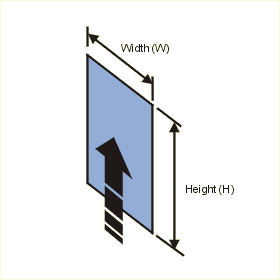Close

This page calculates the convective and radiative heat transfer from an isothermal vertical plate. The average convective heat transfer coefficient is calculated assuming natural convection conditions. The convection calculation automatically switches between laminar and turbulent convection correlations based on Rayleigh number. The plate transfers heat from only one of its sides. It is assumed to be insulated on the other.The convection calculation is based on Nusselt number correlations.

The heat flow (q) from the plate is calculated as:

q = h A x (Tp - Ta)

Where h is the average heat transfer coefficient, A is the area of the plate, Tp is the plate temperature and Ta is the ambient fluid temperature. h is defined as:

h = Nu k / H

Where Nu is the Nusselt Number, k the conductivity of the fluid and H the height of the plate. The Nusselt number is calculated as:

For Laminar Flow - Ra < 109
Nu = 0.68 + (0.670Ra1/4)/[1+(0.492/Pr)9/16]4/9

For Turbulent Flow - Ra is between > 109
Nu = {0.825 + (0.387Ra1/6)/[1+(0.492/Pr)9/16]8/27}2

For the above equations Ra is the Rayleigh number, Gr is the Grashof number, and Pr is the Prandtl number and are calculated using fluid properties as follows:

Ra = (Gr * Pr)
Pr = kinematic viscosity / thermal diffusivity
Gr = gravity x (coefficient of expansion) x (Tp - Ta) x H3 / (kinematic viscosity)2

In addition, you must define the fluid properties at the film temperature Tf defined as follows, except for the density which is defined at the reference temperature of 20 C.

Tf = (Tp + Ta) / 2

The fluid density at the film temperature is automatically calculated from the following relationship based on the perfect gas law:

Rho = Rhoref (Tref +273) / (Tf + 273)

The radiative heat transfer is calculated as follows:

Qrad = Sigma A x E x(Tp4 - Ta4)
where Sigma, the Stefan-Boltzman constant = 5.678e-8
A is the plate area
E is the surface emissivity
Tp and  Ta are the plate and ambient temperatures respectively, in degrees Kelvin.

References

Churchill, S. W. and H. H. S. Chu, Correlating Equations for Laminar and Turbulent Free Convection from a Vertical Plate, Int. J. Heat and Mass Transfer , 18, 1323, 1975

Incropera, De Witt., Fundamentals of Heat and Mass Transfer, 3rd ed., John Wiley & Sons, p543, eq.9.26 & 9.27, 1990.